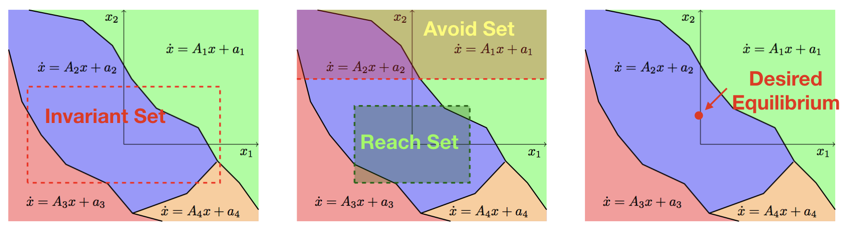Automated Controller Verification and Synthesis

Modern control design leverages numerical optimization to find controllers for continuous-state dynamical systems. The optimization represents a search for valid parameters of a controller, often along with the parameters of a certificate of correctness of that controller.

This work seeks to automate the search for continuous-state controllers and their certificates, with an emphasis on search algorithms with provable behavior. Our approach is to use piece-wise affine functions to model the system dynamics and certificate. This choice makes the conditions simple to derive, with the complexity of the models and certificates controlled by the number of pieces in the functions we use.Example: Verification of Arbitrarily Switching System

We find a piece-wise linear Lyapunov function that certifies asympototic stability of a switched linear system. The system arbitrarily switches between two modes $$\dot x =A_1 x = \left[ \begin{matrix} -5 &-4\\ -1 &-2 \end{matrix} \right] x, \textrm{ and } \dot x = A_2 x = \left[ \begin{matrix} -2 &-4\\ 20 &-2 \end{matrix} \right] x.$$ The differential inclusion $$\dot x \in \mathrm{convexhull} \left(A_1 x , A_2 x\right)$$ captures this arbitrarily switching between the two modes. The red curve in the figure to the right shows a level set of the piece-wise linear Lyapunov function we find, which contains 66 conical pieces. The blue dashed curve is a level set of a polynomial Lyapunov function found using sums-of-squares optimization.